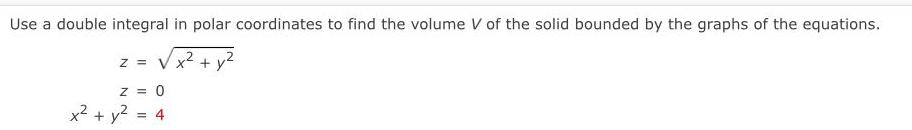Question:

# Use a double integral in polar coordinates to find theUse a double integral in polar coordinates to find the volume V of the solid bounded by the graphs of the equations. z =√x² + y² z = 0 x² + y²= 4